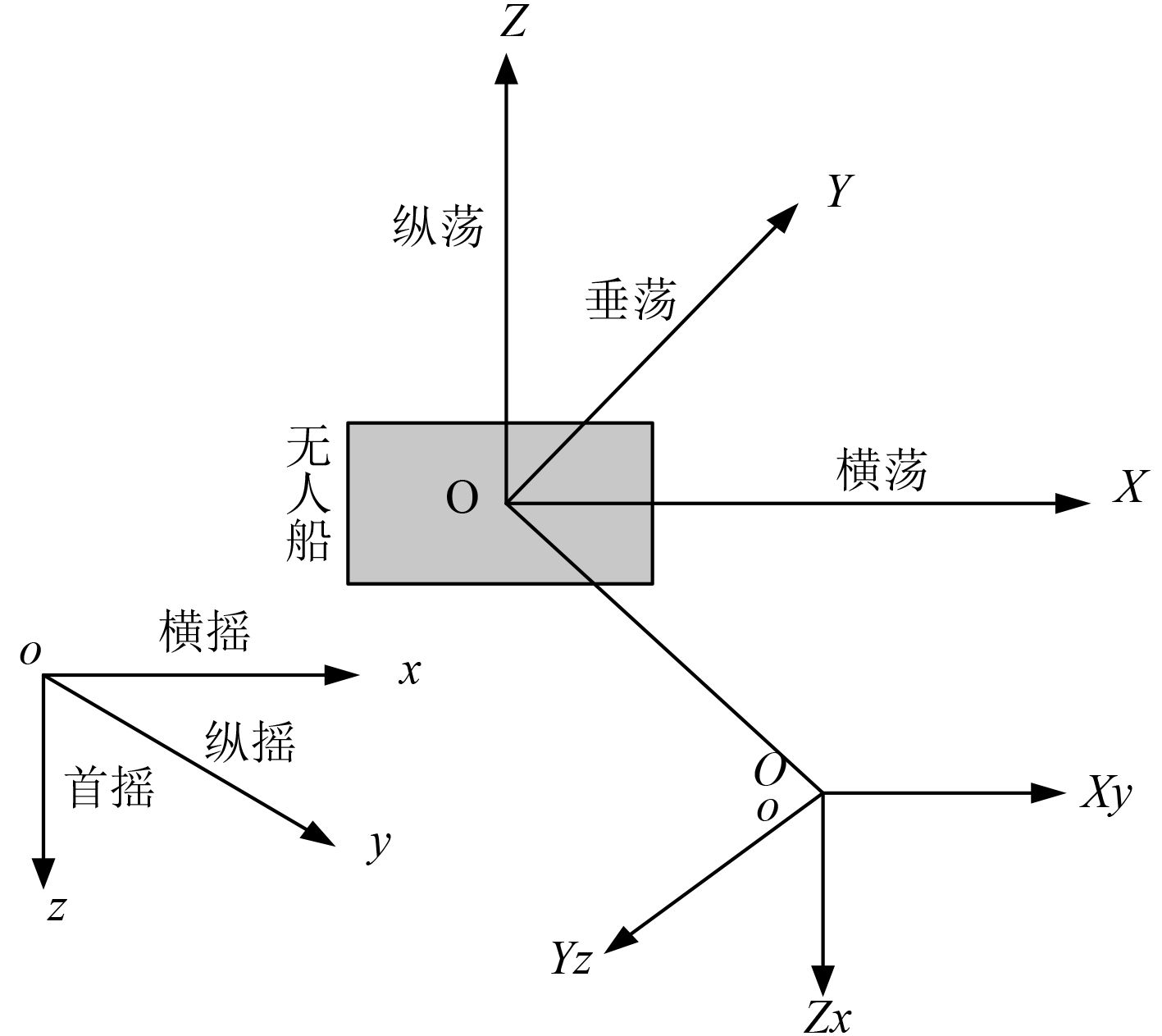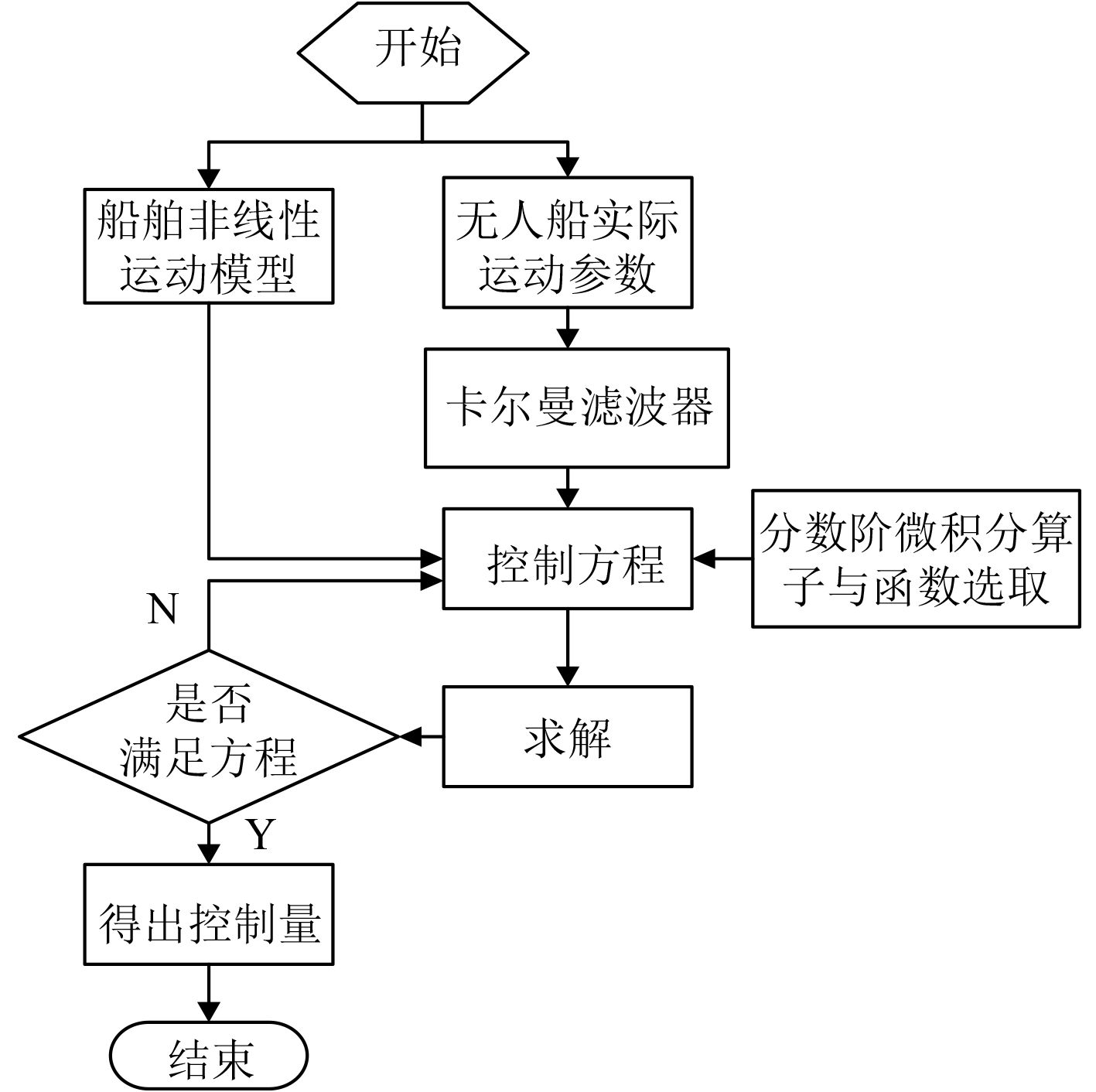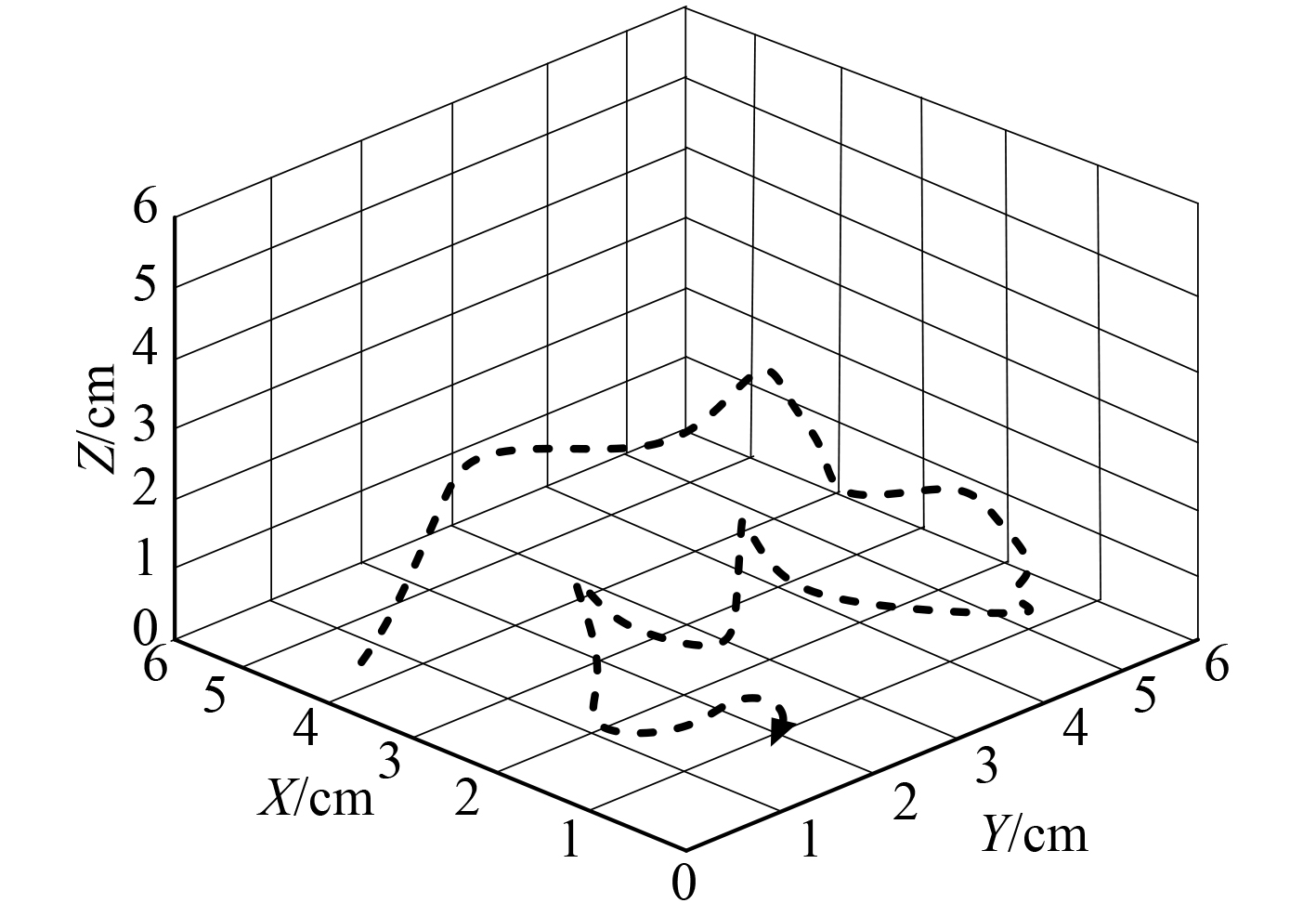﻿ 基于分数阶微积分的无人船运动非线性数学控制模型
 舰船科学技术2022, Vol. 44Issue (7): 78-81    DOI: 10.3404/j.issn.1672-7649.2022.07.015PDF

Nonlinear control of unmanned ship based on Fractional Calculus
ZHAO Xin-yu
School of Mathematics and Information Science of Henan Normal University, Xinxiang 453007, China
Abstract: In case of severe weather such as strong wind and waves, the unmanned ship is easy to be affected and nonlinear motion occurs. If the stability control cannot be implemented in time, it is easy to capsize. To solve the above problems, a nonlinear mathematical control model of unmanned ship motion based on fractional calculus is studied. In this study, six types of nonlinear motions of unmanned ship are described, namely surge, sway, heave, roll, pitch and yaw. Collect ship motion parameters through various equipment to clarify the state of unmanned ship. Combined with fractional calculus function and operator, the nonlinear mathematical control equation of unmanned ship motion is established and solved, the control quantities in three directions of x-axis, y-axis and z-axis are obtained, and the control model is constructed. The results show that compared with the control model based on neural network, the control method based on feedback linearization and fuzzy control system, the model has stronger anti rolling and anti surge effect, and can better ensure the stability of the ship in strong wind and big waves.
Key words: fractional calculus     unmanned ship     motion parameters     nonlinear mathematical control model
0 引　言

1 无人船运动非线性数学控制模型设计

1.1 无人船非线性运动描述表 1 无人船非线性运动描述 Tab.1 Nonlinear motion description of unmanned ship图 1 无人船非线性运动参考坐标系 Fig. 1 Nonlinear motion reference coordinate system of unmanned ship

 $\begin{split} & F\left( {A,A1,A2,B,B1,B2} \right) = \hfill \\ & \frac{{g\left[ \begin{gathered} m\left( {X{\text{ + }}Y + Z} \right) + L\left( {u + v + w} \right) \hfill \\ + m\left( {K + M{\text{ + }}N} \right) + L\left( {p + q + r} \right) \hfill \\ \end{gathered} \right]}}{{m \cdot Q \cdot U}} 。\end{split}$ (1)

1.2 无人船运动参数采集表 2 船舶运动参数采集方式 Tab.2 Acquisition methods of ship motion parameters

1）输入原始含噪无人船运动参数数据为Yi

2）利用主成分分析方法对Yi进行处理，并转换为矩阵的形式，记为R，公式为：

 ${\boldsymbol{R}} = \left[ \begin{array}{*{20}{c}} {r_{11}}&{{r_{12}}}&{...}&{{r_{1n}}} \\ {r_{21}}&{{r_{22}}}&{...}&{{r_{2n}}} \\ ... \\ {r_{m1}}&{{r_{m2}}}&{...}&{{r_{mn}}} \\ \end{array} \right]。$ (2)

3）选择矩阵中前 $k$ 个主成分，并让其保持不变。

4）将除了 $k$ 个主成分外的所有主成分分量转换为二维图像形式，记为 $H$

5）利用K-SVD 算法对 $H$ 进行去噪处理，去噪公式如下：

 $H' = \frac{{KT\sum {{r_{ij}}} }}{{\sqrt m }} 。$ (3)

6）对去噪后的二维图像进行逆变换。

7）得到去噪后的无人船运动参数数据，完成去噪处理。此外，为保证不同数据具有统一性，需要去除其量纲。公式如下：

 $x' = \frac{{x - \phi }}{\varphi } 。$ (4)

1.3 无人船运动非线性数学控制实现

 $\left\{ \begin{gathered} {X_{t + 1}} = \frac{{P_\beta ^\alpha \left( {{V_t} + {C_t}\sqrt L + {G_t}} \right)}}{2}，\hfill \\ {Y_{t + 1}} = \sqrt {\frac{{f\left( {{D_t} \cdot {C_t}} \right)}}{2}} ，\hfill \\ {Z_{t + 1}} = \frac{{P\left( {{K_t} + {M_t} + {N_t}} \right)}}{{pqr}} 。\end{gathered} \right.$ (5)

1）分数阶微积分算子描述为：

 P_\beta ^{\alpha} = \left\{ \begin{aligned} & \dfrac{{{d^\alpha }}}{{d{t^\beta }}}, \alpha > 0，\\ &{1,} {\alpha = 0}，\\ &{\int_a^{\beta} {{{(d\eta )}^{\alpha} }} ,} {{\alpha} < 0} 。\end{aligned} \right. (6)

2）分数阶微积分基本函数描述为：

 $f = \sum\limits_{k = 0}^\infty {\frac{{{B^k}}}{{\lambda (\alpha k + \beta )}}} 。$ (7)图 2 无人船运动非线性数学控制实现过程 Fig. 2 Realization process of nonlinear mathematical control of unmanned ship motion
2 模型测试与分析

2.1 测试环境搭建

2.2 工况相关参数设置表 3 工况相关参数设置 Tab.3 Related parameter settings
2.3 无人船运动控制量图 3 无人船运动控制量 Fig. 3 Motion control quantity of unmanned ship
2.4 控制效果分析表 4 减摇和减荡效果（%） Tab.4 Anti rolling and anti sway effect (%)
3 结　语

  张腾, 任俊生, 梅天龙. 基于傅汝德-克雷洛夫力非线性法的规则波浪中船舶运动数学模型[J]. 交通运输工程学报, 2020, 20(2): 77-87.  张彪, 彭秀艳, 高杰. 基于ELM-EMD-LSTM组合模型的船舶运动姿态预测[J]. 船舶力学, 2020, 24(11): 1413-1421. DOI:10.3969/j.issn.1007-7294.2020.11.005  赵顺利, 李伟, 张文拴. 基于径向基函数神经网络的船舶航迹自抗扰控制[J]. 上海海事大学学报, 2020, 41(4): 20-24.  王书恒, 孟帅, 丁明, 等. 基于反馈线性化的船载自稳平台非奇异滑模控制[J]. 船舶工程, 2021, 43(6): 96-102.  吴正平, 邓聪, 文海. 模糊线性/非线性自抗扰切换控制及其应用[J]. 航空学报, 2021, 42(9): 473-480.  李佳勇. 智能化技术在电气自动化中的运用[J]. 工程技术研究, 2021, 6(13): 35-36. DOI:10.3969/j.issn.1671-3818.2021.13.015  周文俊, 朱仁传, 陈曦, 等. 基于三维非线性区域分割法的船舶运动时域计算研究[J]. 中国造船, 2020, 61(1): 1-17. DOI:10.3969/j.issn.1000-4882.2020.01.001  解骞, 李佳鑫, 贾业萌, 等. 基于非线性动力学的分数阶直驱式永磁同步发电机建模与性能分析[J]. 西安理工大学学报, 2021, 37(3): 441-450.  王莉, 李庭贵, 王旭. 基于李雅普诺夫理论的电液伺服系统非线性位置控制研究[J]. 机床与液压, 2021, 49(9): 136-140. DOI:10.3969/j.issn.1001-3881.2021.09.026  张鑫, 李嘉欣. 基于分数阶微积分的机械臂滑模控制的研究[J]. 系统仿真学报, 2020, 32(5): 911-917.